# Operator¶

class odl.operator.operator.Operator(*args, **kwargs)[source]

Bases: object

Abstract mathematical operator.

An operator is a mapping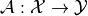between sets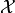(domain) and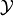(range). The evaluation of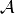at an element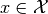is denoted by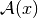and produces an element in: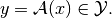Programmatically, these properties are reflected in the Operator class described in the following.

Abstract attributes and methods

Operator is an abstract class, i.e. it can only be subclassed, not used directly.

Any subclass of Operator must have the following attributes:

domainSet

The set of elements this operator can be applied to

rangeSet

The set this operator maps to

It is highly recommended to call super(MyOp, self).__init__(domain, range) in the __init__() method of any subclass MyOp, where domain and range are the arguments specifying domain and range of the new operator. In that case, the attributes Operator.domain and Operator.range are automatically provided by the parent class Operator.

In addition, any subclass must implement the private method Operator._call(). It signature determines how it is interpreted:

In-place-only evaluation: _call(self, x, out[, **kwargs])

In-place evaluation means that the operator is applied, and the result is written to an existing element out provided, i.e.

_call(self, x, out)  <==>  out <-- operator(x)

Parameters:

xOperator.domain element

An object in the operator domain to which the operator is applied

outOperator.range element

An object in the operator range to which the result of the operator evaluation is written.

Returns:

None (return value is ignored)

Out-of-place-only evaluation: _call(self, x[, **kwargs])

Out-of-place evaluation means that the operator is applied, and the result is written to a new element which is returned. In this case, a subclass has to implement the method

_call(self, x)  <==>  operator(x)

Parameters:

xOperator.domain element

An object in the operator domain to which the operator is applied

Returns:

out

An object in the operator range holding the result of the operator evaluation

Dual-use evaluation: _call(self, x, out=None[, **kwargs])

Evaluate in-place if out is given, otherwise out-of-place.

Parameters:

xOperator.domain element

An object in the operator domain to which the operator is applied

outOperator.range element, optional

An object in the operator range to which the result of the operator evaluation is written

Returns:

None (return value is ignored)

Notes

Attributes
adjoint

domain

Set of objects on which this operator can be evaluated.

inverse

Return the operator inverse.

is_functional

True if this operator’s range is a Field.

is_linear

True if this operator is linear.

range

Set in which the result of an evaluation of this operator lies.

Methods

 _call(self, x[, out]) Implementation of the operator evaluation. derivative(self, point) Return the operator derivative at point. norm(self[, estimate]) Return the operator norm of this operator.
__init__(self, domain, range, linear=False)[source]

Initialize a new instance.

Parameters
domainSet

The domain of this operator, i.e., the set of elements to which this operator can be applied

rangeSet

The range of this operator, i.e., the set this operator maps to

linearbool, optional

If True, the operator is considered as linear. In this case, domain and range have to be instances of LinearSpace, or Field.